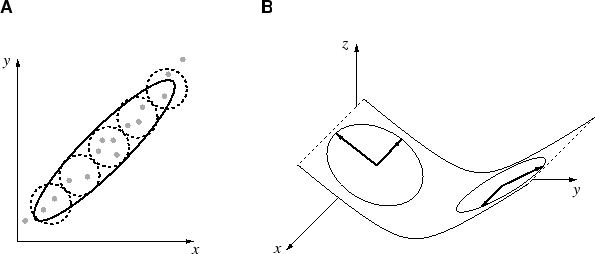Next: 3.2 Extension of Neural Up: 3. Mixture of local Previous: 3. Mixture of local

# 3.1 Motivation for local PCA

When describing a data distribution with a mixture model the question arises what kind of units should the mixture contain. The simplest local description is a point, the second simplest a linear model, which can be obtained from a local PCA. In a Gaussian density model of the data points, a point corresponds to a uniform Gaussian function, and a local PCA corresponds to a multivariate Gaussian (section 2.3.1). Thus, the local iso-density surface is a sphere respective an ellipsoid. Therefore, the decision between points and local PCA can be also regarded as a decision between spheres and ellipsoids. Despite its greater complexity, local PCA is favorable over a point for the following reasons. An ellipsoid can describe a local structure for which many spheres are needed (figure 3.1.A). Furthermore, sensorimotor distributions are usually constrained locally to subspaces with fewer dimensions than the space of the training data. Thus, directions exist in which locally the distribution has zero variance (or almost zero because of noise). PCA can omit directions of zero variance; a point cannot (figure 3.1.B). Using local PCA also helps to cope with the problem of noise dimensions as mentioned in section 1.5.5. An ellipsoid can extend with one of its principal components into the additional noise dimension; the number of points needed to take care of the additional variance increases over-proportionally (compare figure 3.1 with figure 1.9).Next: 3.2 Extension of Neural Up: 3. Mixture of local Previous: 3. Mixture of local
Heiko Hoffmann
2005-03-22